About Qweas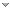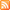RSS Feeds     BBS Forum Make Qweas.com My Home Page     Bookmark this page Register     Login     Help     Send Feedback
 All software Windows Mac Linux Mobile Games Screensavers Source Code Webware
Windows   Mac   Linux   Mobile   Games   Screensavers
 Audio/Video Business Communication Desktop Develop Education Games Graphic Home Network Security Servers System Web Computer , Dictionaries , Geography , Kids , Languages , Mathematics , Other , Reference Tools , Science , Teaching & Training Tools
 Recommends 4Media Video Converter Platinum for Mac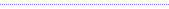ABC Amber Lotus 1-2-3 ConverterYouTube2DVD BurnerPhoto DVD Maker ProfessionalFlipSmartVideo Converter for MacZiperelloLiong: The Dragon DanceAV Music MorpherBitTorrent Acceleration PatchZC RM RMVB to DVD CreatorTowers of Hanoi for Pocket PC

 Related Reviews - more gif optimizerphoto optimizersystem optimizernetwork optimizersdatabase optimizationsearch engine optimizesearch engine optimizeroptimize connection speedgraphic convertersanimation gif builderanimation gif converterflash video converterconvert video to picturesbatch image converterconvert image to iconconvert pdf to imagemathematics softwaremathematics software for kidsmathematics teaching softwaremathematical games
Home > Education > Mathematics > Visual Optim > Publisher

Visual Optim Publisher

Visual Optim is a math program for linear and nonlinear programming.

Publisher: GraphNow Software

Website: http://www.graphnow.com/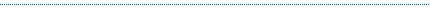GraphNow Software develops: Visual Probability : Distribution computing and graphing. ; Visual Fractal : Create Mandelbrot fractal, Julia fractal etc ; Visual Calculus : Calculus grapher for calculus teaching. ; Visual Stats : Statistics software for data analysis. ; Visual Matrix : matrix calculator implement matrix operations ...Visual Complex

Visual Complex is a graph software to create graph of complex function. 3D function graphs and 2D color maps can be created with this grapher. It is a graphing calculator to create a graph of complex function.Visual Optim

Visual Optim is a math program for one dimension searching, linear programming, unconstrained nonlinear programming and constrained nonlinear programming. Visual Optim features include: One dimensional searching, Linear programming, and much more...Visual Calculus

Visual Calculus is an easy-to-use calculus grapher for Graphing limit, derivative function, integral, 3D vector, series, ode etc. Visual Calculus can create 2D, 2.5D and 3D function graphs, animations and table graphs.Visual Stats

Visual Probability is a software to compute and graph probability density function values, cumulative distribution function values, quantile values, means and variances of ten more distributions. visualizaton.Visual Matrix

Visual Matrix is a matrix calculator to implement matrix operations and matrix decompositions. The features are including: Basic matrix operations: +, -, .*, *, ', determinant, etc.; Matrix decompositions: LU decomposition, QR decomposition.Visual Fitting

Math tool for linear, nonlinear curve fitting and surface fitting, curve and surface can be created. It can implement curve fitting and graphing of linear and nonlinear models, can implement surface fitting and graphing of any binary function models.Visual Fractal

Visual Fractal is an interesting grapher to create a graph of fractal. With this interesting fractal software, you can use Newton's method to solve a complex equation and show the fractal graph in the plot areaVisual Probability

Visual Probability is a software to compute and graph probability density function values, cumulative distribution function values, quantile values, means and variances of ten more distributions. visualizaton.

TAGS OF VISUAL OPTIM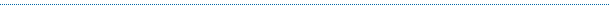Site Map | Sort by Letters | Submit Software | Popular Downloads | Editor Picks | New Releases : Mac , Freeware | Updates : Mac , Freeware